# Plotting Libaries Overview¶

### Email: [email protected]¶

You may need to install some libraries first for this notebook to run smoothly. Edit the commands below to the subset of libraries you need to install and execute it. Skip it if you have all of these.

In [ ]:
!pip install six, pytz, dateutil, Flask, Redis
!pip install numpy, scipy, statsmodels, patsy, pandas, networkx
!pip install matplotlib, mpld3, seaborn, bokeh, rpy2

#git clone https://github.com/vispy/vispy.git
#cd vispy
#python setup.py install


# Matplotlib:¶

Matplotlib provides a stateful scripting interface for generating graphics similar to MATLAB's syntax and appearance. Matplotlib renders to a "back end", which is usually a raster graphics canvas. The strength of this approach is that, once rendered, the data loads into a web page as an image and is therefore very fast. For ipython notebooks with lots of plots, this is the way to go because web browsers are optimized for displaying tons of images.

Can be used to:

• plot interactively from python shell and pop up windows from commandline
• embed into GUI to build rich apps
• batch generate postscript images from numerical simulations
• dynamically serve up graphs in a web app

Primary use case: Very nice "publication" quality plots with customized look, typefaces, and annotations, that can be exported to pdf.

Strengths: Very widely adopted, very flexible, tight integration with iPython notebook, great for making presentation quality graphs and figures.

Weaknesses: Uses MATLAB-inspired plotting syntax, renders things slowly without GPU acceleration, steep learning curve, have to specify/customize a lot of variables to make it look good, and the defaults are pretty terrible.

Matplotlib Example Gallery: http://matplotlib.org/gallery.html

In :
%pylab inline
import numpy as np
import pandas as pd
matplotlib.rcParams['figure.figsize'] = 15, 5 #set default image size for this interactive session
matplotlib.rcParams.update({'font.size': 16, 'font.family': 'serif'}) #update the matplotlib configuration parameters

Populating the interactive namespace from numpy and matplotlib

In :
x = linspace(0, 5, 10)
y = x ** 2
fig, ax1 = subplots()

ax1.plot(x, x**2, lw=2, color="blue", label="test")
ax1.set_ylabel(r"area $(m^2)$", fontsize=18, color="blue")
for label in ax1.get_yticklabels():
label.set_color("blue")

ax2 = ax1.twinx()
ax2.plot(x, x**3, lw=2, color="red", label="test")
ax2.set_ylabel(r"volume $(m^3)$", fontsize=18, color="red")
for label in ax2.get_yticklabels():
label.set_color("red")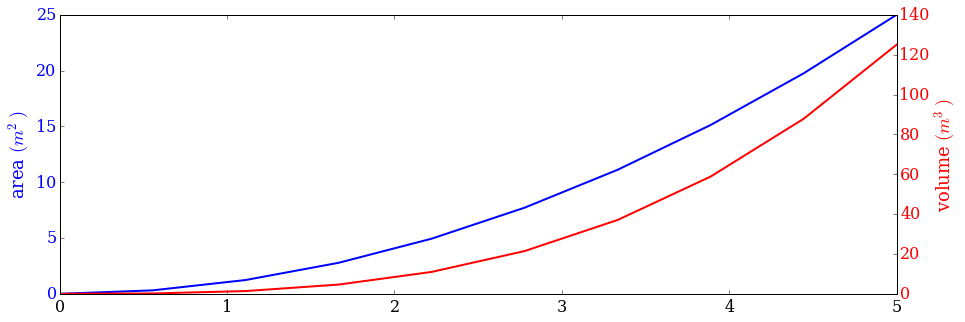In :
n = array([0,1,2,3,4,5])
xx = np.linspace(-0.75, 1., 100)

In :
fig, axes = subplots(1, 4, figsize=(15,5))

axes.scatter(xx, xx + 0.25*randn(len(xx)), label="scatter")
axes.step(n, n**2, lw=2, label="step")
axes.bar(n, n**2, align="center", width=0.5, alpha=0.5, label="bar")
axes.fill_between(x, x**2, x**3, color="green", alpha=0.5);

axes.legend(loc=2); # upper left corner
axes.legend(loc=2);
axes.legend(loc=2);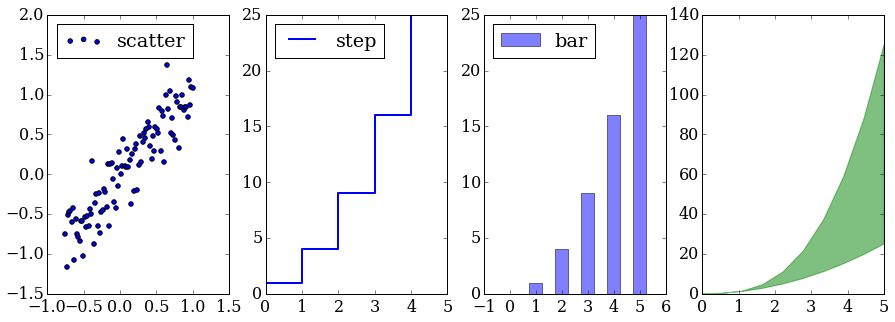# Interactive Widgets¶

IPython has recently introduced interactive widgets that tie together Python code running in the kernel and JavaScript/HTML/CSS running in the browser. Just like manipulate command in Mathematica, it works by repeatedly calling the function that renders the graphics with the new variable values, generating a new image on each iteration. This can be slow.

In :
from IPython.html.widgets import interact
import networkx as nx

matplotlib.rcParams['figure.figsize'] = 5, 5

In :
# wrap a few graph generation functions so they have the same signature

def random_lobster(n, m, k, p):
return nx.random_lobster(n, p, p / m)

def powerlaw_cluster(n, m, k, p):
return nx.powerlaw_cluster_graph(n, m, p)

def erdos_renyi(n, m, k, p):
return nx.erdos_renyi_graph(n, p)

def newman_watts_strogatz(n, m, k, p):
return nx.newman_watts_strogatz_graph(n, k, p)

def plot_random_graph(n, m, k, p, generator):
g = generator(n, m, k, p)
nx.draw(g)
plt.show()

In :
interact(plot_random_graph, n=(2,30), m=(1,10), k=(1,10), p=(0.0, 1.0, 0.001),
generator={'lobster': random_lobster,
'power law': powerlaw_cluster,
'Newman-Watts-Strogatz': newman_watts_strogatz,
u'Erdős-Rényi': erdos_renyi,
});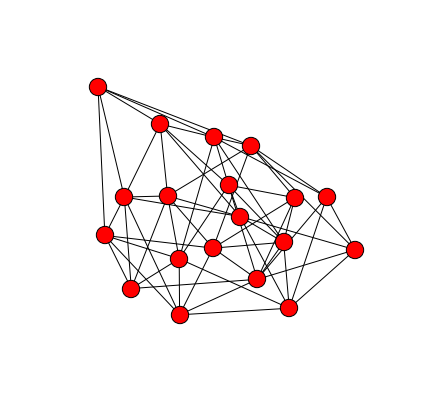To save publication quality figures, we use the pdf backend to matplotlib, and generate our figure as a vector graphic rather than the raster graphic image. We can call pdf.savefig() multiple times and it will save multiple pages to the pdf filename specified.

In :
from matplotlib.backends.backend_pdf import PdfPages

with PdfPages('polarplot.pdf') as pdf:
# polar plot using add_axes and polar projection
fig = plt.figure(figsize=(5,5))
ax = fig.add_axes([0.0, 0.0, .6, .6], polar=True)
t = linspace(0, 2 * pi, 100)
ax.plot(t, t, color='blue', lw=3);
pdf.savefig(fig)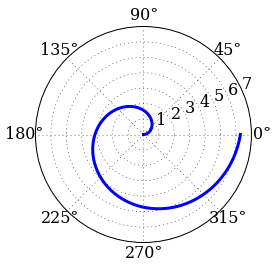# Seaborn:¶

Seaborn is a library for making attractive and informative statistical graphics in Python. It is built on top of matplotlib and tightly integrated with the PyData stack, including numpy, pandas data structures, and statistical routines from scipy and statsmodels. It offers nice color palettes and simple built-in defaults for statistical plots, so not much tweaking is needed compared to matplotlib to get a sophisticated result.

Strengths: well-suited to statistical plots (like those in R). Can be used to "freshen up" the look of matplotlib plots. Runs on top of matplotlib, so you get all of that functionality as well, including exporting to vector graphic pdfs.

Weaknesses: Relatively new codebase, API is still emerging / evolving. Has most of the same limitations as matplotlib in terms of rendering speed.

In :
import seaborn as sns
from scipy import stats, optimize

In :
#Diverging: useful when data has natural, meaningful break-point
#like correlation values which are spread around zero
sns.palplot(sns.color_palette("coolwarm", 7))In :
#Qualitative: useful when data is categorical
sns.palplot(sns.color_palette("Set2", 10))Sequential: useful when data range from 'low' to 'high' values The cubehelix color palette system makes sequential palettes with a linear increase or decrease in brightness and some variation in hue. This means that the information in your colormap will be preserved when converted to black and white (for printing) or when viewed by a colorblind individual. Matplotlib has the default cubehelix version built into it:

In :
sns.palplot(sns.color_palette("cubehelix", 8))Seaborn adds an interface to the cubehelix system so that you can make a variety of palettes that all have a well-behaved linear brightness ramp. The default palette returned by the seaborn cubehelix_palette() function is a bit different from the matplotlib default in that it does not rotate as far around the hue wheel or cover as wide a range of intensities. It also reverses the order so that more important values are darker:

In :
sns.palplot(sns.cubehelix_palette(8, start=2))One of my favorite functions within Seaborn is a joint distribution plot that allows visualization of a bivariate distribution and its marginals.

This is similar to a histogram, except instead of coding the number of observations in each bin with a position on one of the axes, it uses a color-mapping to give the plot three quantitative dimensions.

In :
x = stats.gamma(3).rvs(5000)
y = stats.gamma(5).rvs(5000)
with sns.axes_style("white"):
sns.jointplot(x, y, kind="hex", color="#4CB391"); #change kind="reg" to show regression line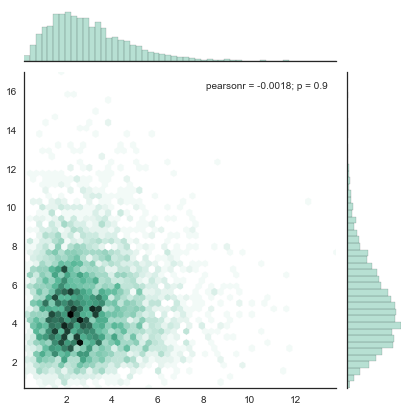Another great statistical plot, the violin plot conveys the same information as a boxplot, showing the median, and 25th and 75th percentiles, adding the shape of the distribution. And we can add the individual observations as well in two different ways:

In :
d1 = stats.norm(0, 5).rvs(100)
d2 = np.concatenate([stats.gamma(4).rvs(50),
-1 * stats.gamma(4).rvs(50)])
data = pd.DataFrame(dict(d1=d1, d2=d2))
data = pd.melt(data.ix[:50], value_name="y", var_name="group")
f, (ax_l, ax_r) = plt.subplots(1, 2)
sns.violinplot(data.y, data.group, "points", positions=[1, 2], color="RdBu", ax=ax_l)
sns.violinplot(data.y, data.group, "stick", positions=[3, 4], color="PRGn", ax=ax_r)
plt.tight_layout()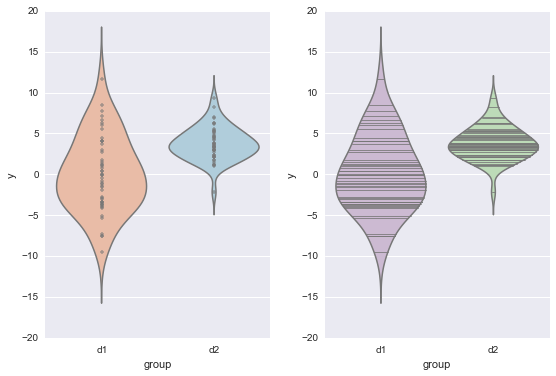Seaborn switches many graphics defaults that will affect other graphics from libraries based on matplotlib, like mpld3 below. We need to reset the defaults with the following command.

In [ ]:
sns.reset_orig


# mpld3:¶

mpld3 is a package allowing seamless visualization of matplotlib plots using D3js javascript renderer. D3js is a popular Javascript library for interactive data visualizations for the web. This means you can use the same syntax as within matplotlib as well as add custom javascript plugins for interactivity and then view your graphics within the browser, a webpage or IPython. Since your figure is now an HTML Canvas object, you can benefit from GPU acceleration.

Figures can be saved to file as stand-alone HTML format (save_html()), or as JSON format (save_json() note that custom plugins which are not built into mpld3 will not be part of the JSON serialization).

Strengths: familiar matplotlib syntax, instantly turn any matplotlib graphic into an HTML Canvas object and add interactivity, can have GPU acceleration

Weaknesses: need familiarity with Javascript to add most interactivity, can only export graphics for the web

In :
import mpld3
from mpld3 import plugins, utils


For example, here is the built-in Linked Brushing plugin that allows exploration of multi-dimensional datasets. Selecting points with the brush lets you quickly explore the relationships between the points in many different 2D projections.

In :
fig, ax = plt.subplots(3, 3, figsize=(6, 6))
ax = ax[::-1]

X = np.random.normal(size=(3, 100))
for i in range(3):
for j in range(3):
ax[i, j].xaxis.set_major_formatter(plt.NullFormatter())
ax[i, j].yaxis.set_major_formatter(plt.NullFormatter())
points = ax[i, j].scatter(X[j], X[i])

mpld3.display()

Out:

My next example is borrowed from the Pythonic Perambulations blog. Here we can hover over points in our scatterplot to see the associated sinusoid above. This is clearly a bit more complicated, requiring knowledge of Javascript to create a custom plugin for the interactivity.

Use the toolbar buttons at the bottom-right of the plot to enable zooming and panning, and to reset the view.

In :
class LinkedView(plugins.PluginBase):
"""A simple plugin showing how multiple axes can be linked"""

JAVASCRIPT = """
mpld3.Plugin.call(this, fig, props);
};

var pts = mpld3.get_element(this.props.idpts);
var line = mpld3.get_element(this.props.idline);
var data = this.props.data;

function mouseover(d, i){
line.data = data[i];
line.elements().transition()
.attr("d", line.datafunc(line.data))
.style("stroke", this.style.fill);
}
pts.elements().on("mouseover", mouseover);
};
"""

def __init__(self, points, line, linedata):
if isinstance(points, matplotlib.lines.Line2D):
suffix = "pts"
else:
suffix = None

"idpts": utils.get_id(points, suffix),
"idline": utils.get_id(line),
"data": linedata}

fig, ax = plt.subplots(2)

# scatter periods and amplitudes
np.random.seed(0)
P = 0.2 + np.random.random(size=20)
A = np.random.random(size=20)
x = np.linspace(0, 10, 100)
data = np.array([[x, Ai * np.sin(x / Pi)]
for (Ai, Pi) in zip(A, P)])
points = ax.scatter(P, A, c=P + A,
s=200, alpha=0.5)
ax.set_xlabel('Period')
ax.set_ylabel('Amplitude')

# create the line object
lines = ax.plot(x, 0 * x, '-w', lw=3, alpha=0.5)
ax.set_ylim(-1, 1)

ax.set_title("Hover over points to see lines")

# transpose line data and add plugin
linedata = data.transpose(0, 2, 1).tolist()

mpld3.display()

Out:

# Bokeh:¶

Bokeh is an interactive web visualization library for Python with a modern "grammar for graphics". It provides d3-like html canvas graphics for large or streaming datasets, all without requiring any knowledge of Javascript. Bokeh makes it really fun and easy to interactively explore your data.

Bokeh renders vector graphics directives to an intermediate representation that it sends over a communications socket to the javascript interpreter in your web browser. Then, there is a javascript layer (called BokehJS) that unserializes the JSON and draws it into the HTML5 canvas.

Strengths: uses a modern "visual grammar" for programming graphics, uses the HTML5 canvas object and thus has GPU acceleration in modern browsers, plots can be interactive and easier to explore.

Weaknesses: API and examples are still evolving

In :
from __future__ import division
from collections import OrderedDict
from six.moves import zip
from bokeh.plotting import *
from bokeh.objects import Range1d, ColumnDataSource, HoverTool
from bokeh.sampledata.unemployment1948 import data
output_notebook()